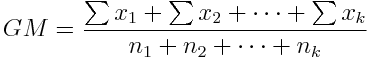The weighted mean, in the context that you need to remember here, is like the "mean mean." If there are several groups of different size, and you want to find the overall mean of all the scores considered as one big group, often called the "grand mean," the calculation would be done as follows:Notice that the grand mean is "en-one times mean one plus en-two times mean two plus en-three times mean three, and so on, divided by the total N. Why is this true (in an arithmetic sense, that is)? Return To Main Page